Скачать презентацию ECE 4371 Fall 2017 Introduction to Telecommunication Engineering Telecommunication

75147ff3c9a34b32fee0ae84d250c34d.ppt

• Количество слайдов: 32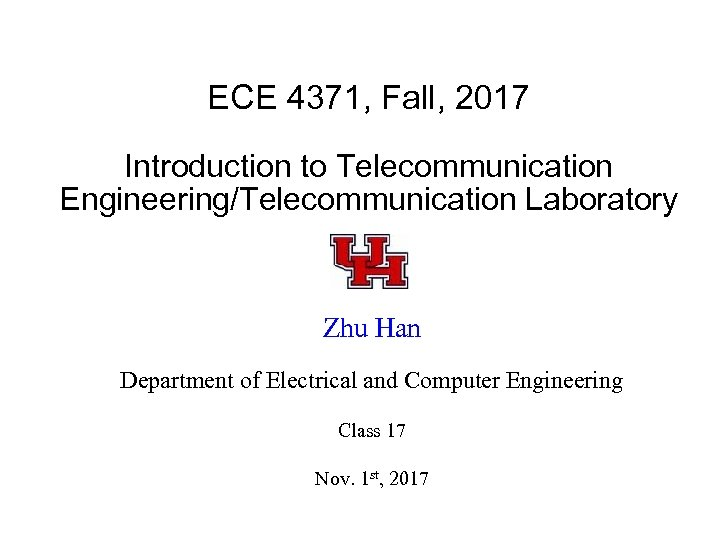ECE 4371, Fall, 2017 Introduction to Telecommunication Engineering/Telecommunication Laboratory Zhu Han Department of Electrical and Computer Engineering Class 17 Nov. 1 st, 2017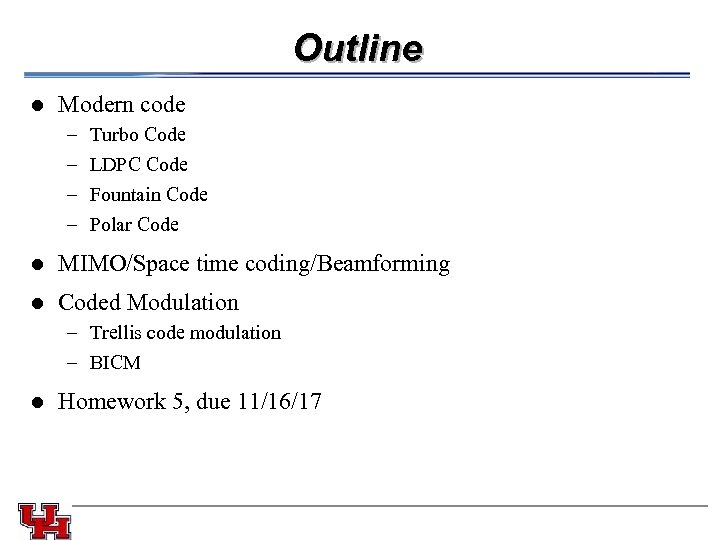Outline l Modern code – – l Turbo Code LDPC Code Fountain Code Polar Code MIMO/Space time coding/Beamforming l Coded Modulation – Trellis code modulation – BICM l Homework 5, due 11/16/17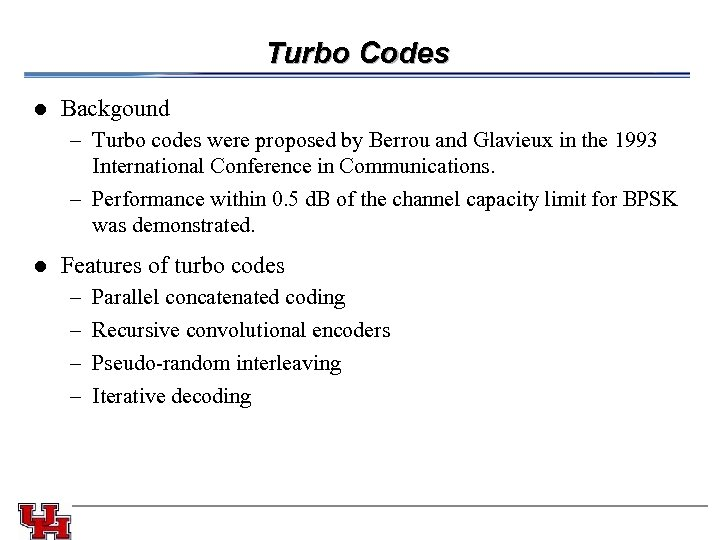Turbo Codes l Backgound – Turbo codes were proposed by Berrou and Glavieux in the 1993 International Conference in Communications. – Performance within 0. 5 d. B of the channel capacity limit for BPSK was demonstrated. l Features of turbo codes – – Parallel concatenated coding Recursive convolutional encoders Pseudo-random interleaving Iterative decoding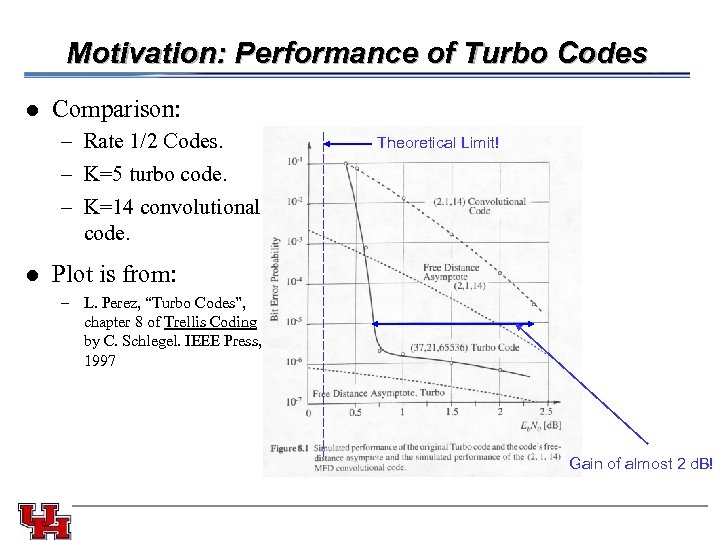Motivation: Performance of Turbo Codes l Comparison: – Rate 1/2 Codes. – K=5 turbo code. – K=14 convolutional code. Theoretical Limit! l Plot is from: – L. Perez, “Turbo Codes”, chapter 8 of Trellis Coding by C. Schlegel. IEEE Press, 1997 Gain of almost 2 d. B!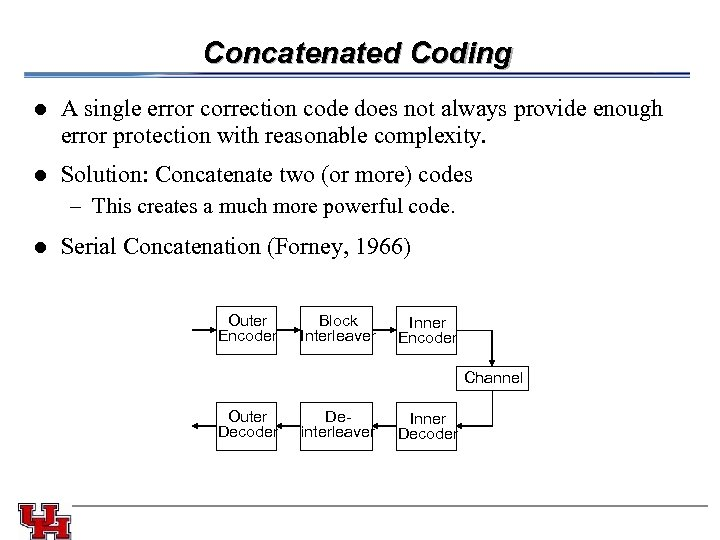Concatenated Coding l A single error correction code does not always provide enough error protection with reasonable complexity. l Solution: Concatenate two (or more) codes – This creates a much more powerful code. l Serial Concatenation (Forney, 1966) Outer Encoder Block Interleaver Inner Encoder Channel Outer Decoder Deinterleaver Inner Decoder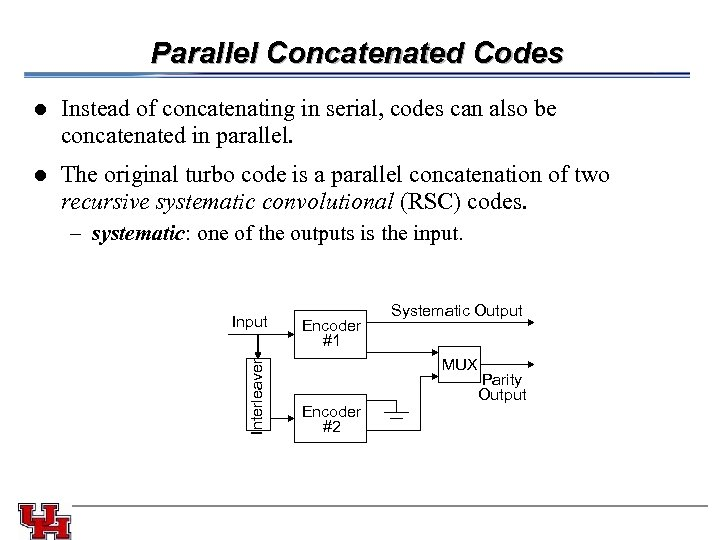Parallel Concatenated Codes l l The original turbo code is a parallel concatenation of two recursive systematic convolutional (RSC) codes. – systematic: one of the outputs is the input. Input Interleaver Instead of concatenating in serial, codes can also be concatenated in parallel. Encoder #1 Systematic Output MUX Encoder #2 Parity Output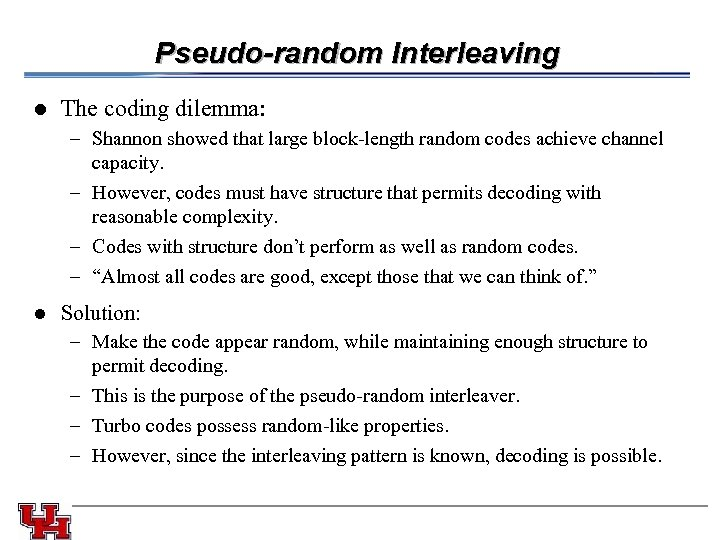Pseudo-random Interleaving l The coding dilemma: – Shannon showed that large block-length random codes achieve channel capacity. – However, codes must have structure that permits decoding with reasonable complexity. – Codes with structure don’t perform as well as random codes. – “Almost all codes are good, except those that we can think of. ” l Solution: – Make the code appear random, while maintaining enough structure to permit decoding. – This is the purpose of the pseudo-random interleaver. – Turbo codes possess random-like properties. – However, since the interleaving pattern is known, decoding is possible.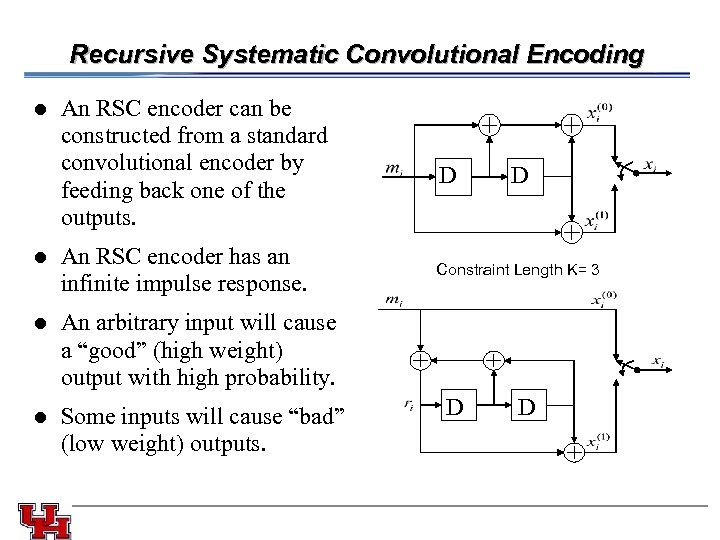Recursive Systematic Convolutional Encoding l l An RSC encoder can be constructed from a standard convolutional encoder by feeding back one of the outputs. An RSC encoder has an infinite impulse response. D D Constraint Length K= 3 l An arbitrary input will cause a “good” (high weight) output with high probability. l Some inputs will cause “bad” (low weight) outputs. D D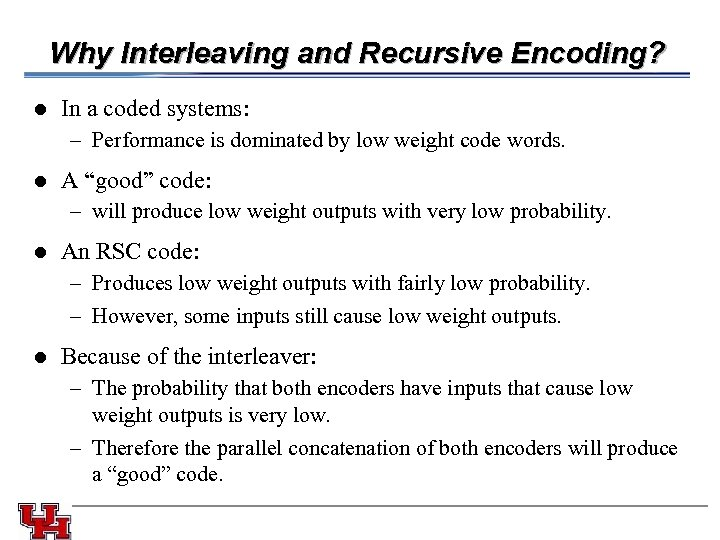Why Interleaving and Recursive Encoding? l In a coded systems: – Performance is dominated by low weight code words. l A “good” code: – will produce low weight outputs with very low probability. l An RSC code: – Produces low weight outputs with fairly low probability. – However, some inputs still cause low weight outputs. l Because of the interleaver: – The probability that both encoders have inputs that cause low weight outputs is very low. – Therefore the parallel concatenation of both encoders will produce a “good” code.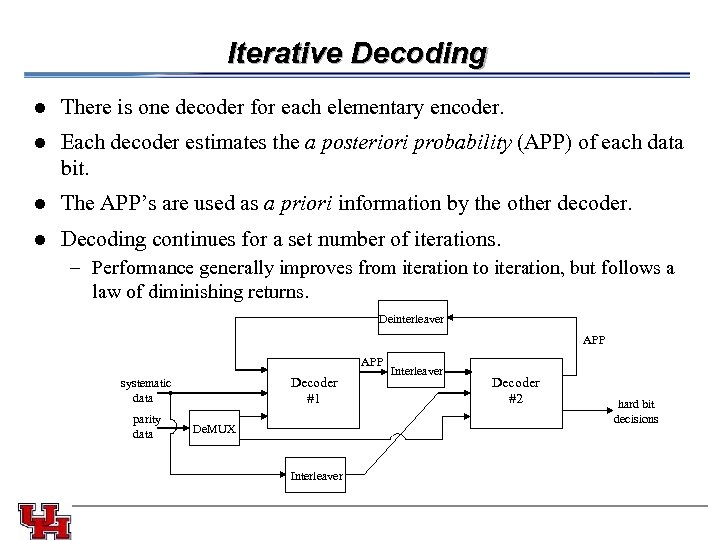Iterative Decoding l l Each decoder estimates the a posteriori probability (APP) of each data bit. l There is one decoder for each elementary encoder. The APP’s are used as a priori information by the other decoder. l Decoding continues for a set number of iterations. – Performance generally improves from iteration to iteration, but follows a law of diminishing returns. Deinterleaver APP Decoder #1 systematic data parity data De. MUX Interleaver Decoder #2 hard bit decisions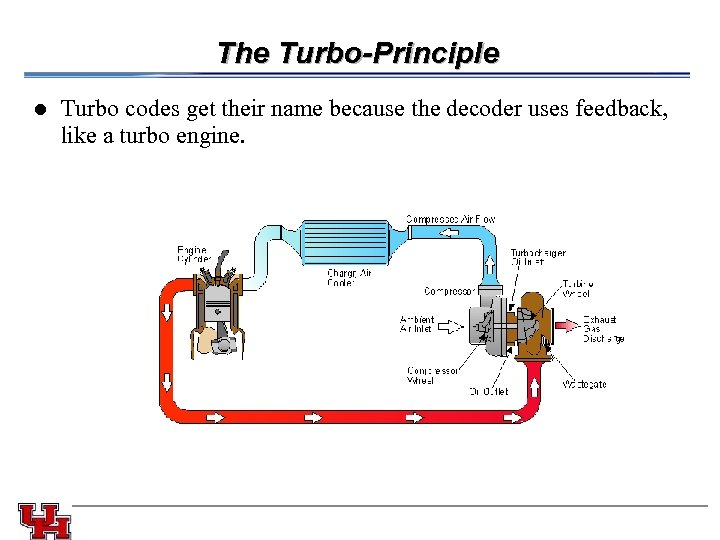The Turbo-Principle l Turbo codes get their name because the decoder uses feedback, like a turbo engine.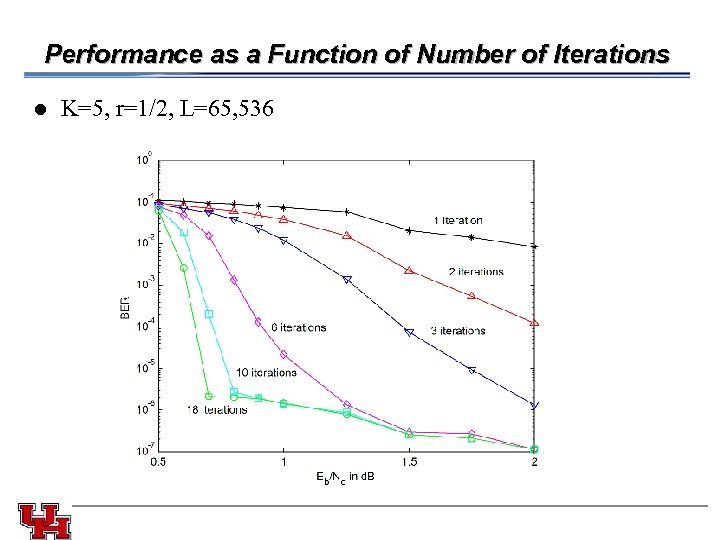Performance as a Function of Number of Iterations l K=5, r=1/2, L=65, 536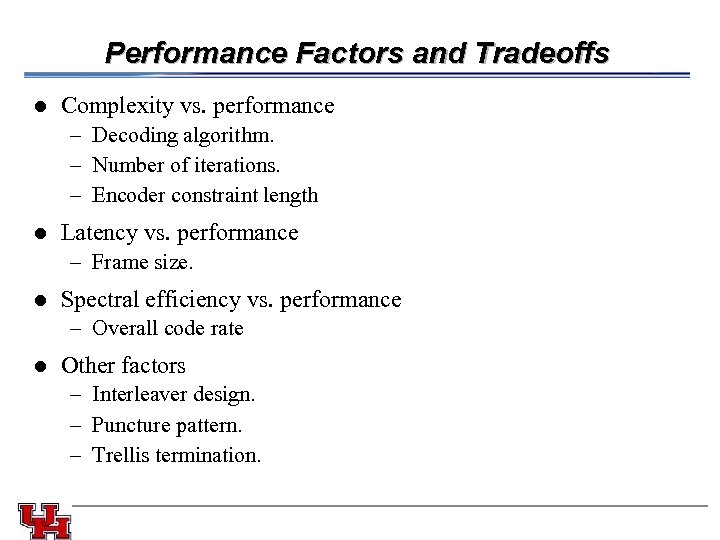Performance Factors and Tradeoffs l Complexity vs. performance – Decoding algorithm. – Number of iterations. – Encoder constraint length l Latency vs. performance – Frame size. l Spectral efficiency vs. performance – Overall code rate l Other factors – Interleaver design. – Puncture pattern. – Trellis termination.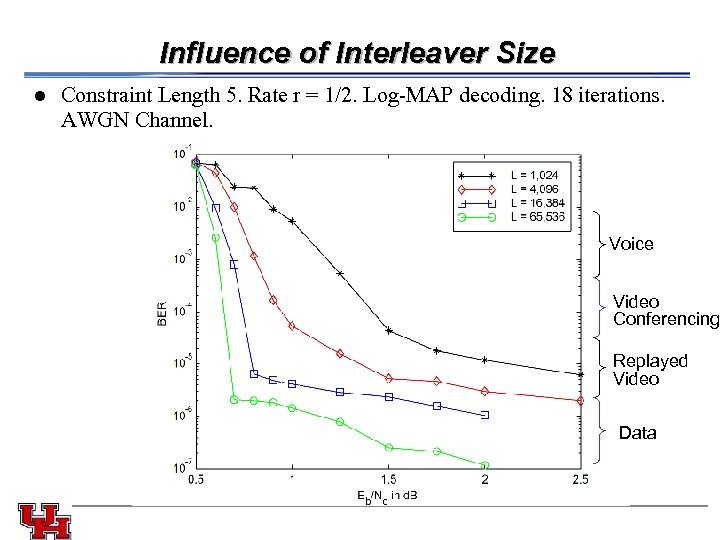Influence of Interleaver Size l Constraint Length 5. Rate r = 1/2. Log-MAP decoding. 18 iterations. AWGN Channel. Voice Video Conferencing Replayed Video Data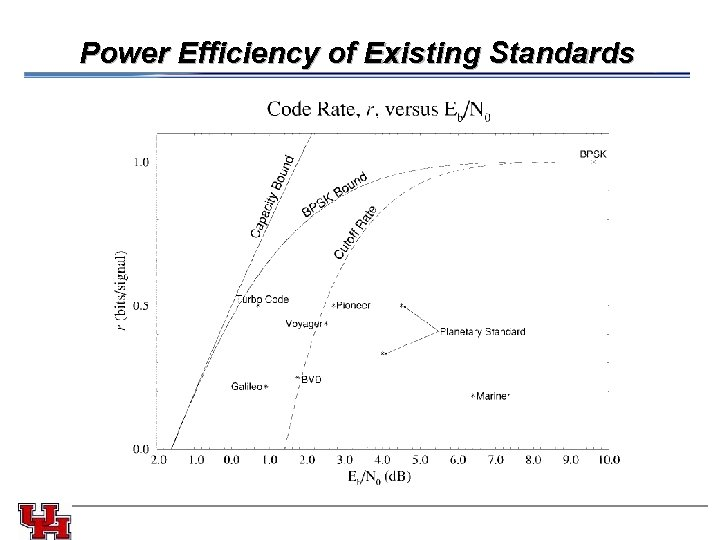Power Efficiency of Existing Standards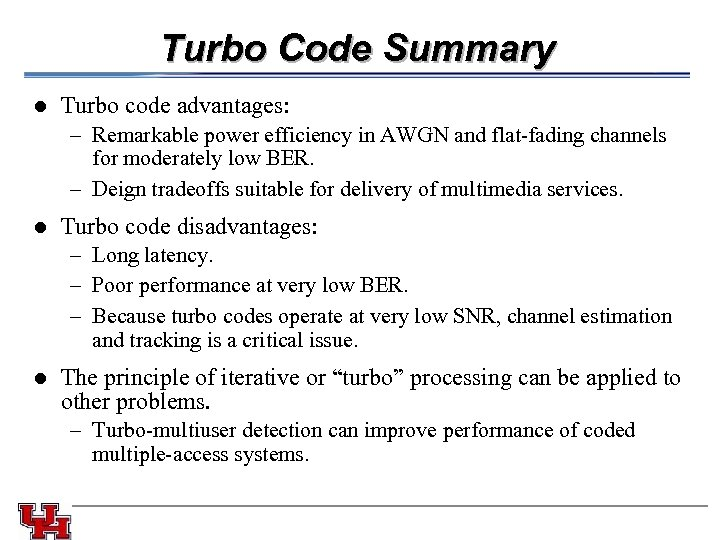Turbo Code Summary l Turbo code advantages: – Remarkable power efficiency in AWGN and flat-fading channels for moderately low BER. – Deign tradeoffs suitable for delivery of multimedia services. l Turbo code disadvantages: – Long latency. – Poor performance at very low BER. – Because turbo codes operate at very low SNR, channel estimation and tracking is a critical issue. l The principle of iterative or “turbo” processing can be applied to other problems. – Turbo-multiuser detection can improve performance of coded multiple-access systems.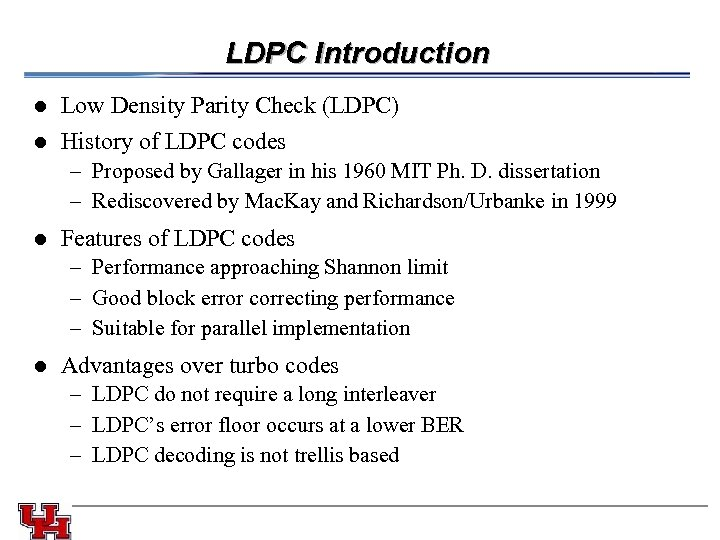LDPC Introduction l l Low Density Parity Check (LDPC) History of LDPC codes – Proposed by Gallager in his 1960 MIT Ph. D. dissertation – Rediscovered by Mac. Kay and Richardson/Urbanke in 1999 l Features of LDPC codes – Performance approaching Shannon limit – Good block error correcting performance – Suitable for parallel implementation l Advantages over turbo codes – LDPC do not require a long interleaver – LDPC’s error floor occurs at a lower BER – LDPC decoding is not trellis based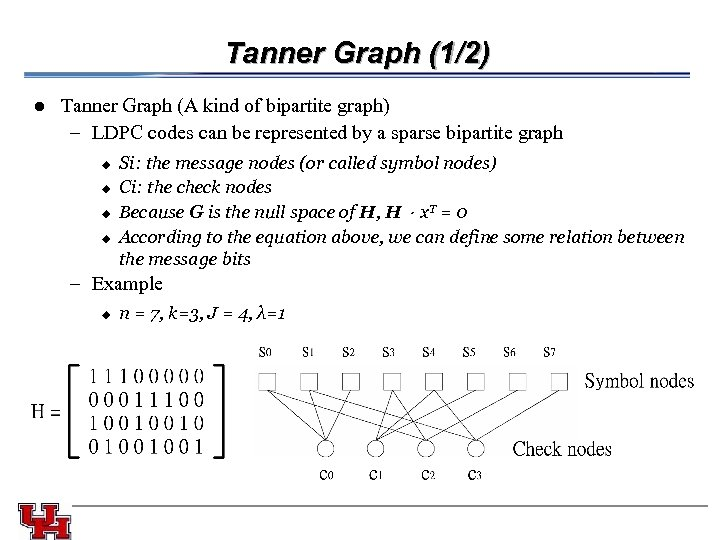Tanner Graph (1/2) l Tanner Graph (A kind of bipartite graph) – LDPC codes can be represented by a sparse bipartite graph u u u u Si: the message nodes (or called symbol nodes) Ci: the check nodes Because G is the null space of H, H．x. T = 0 According to the equation above, we can define some relation between the message bits – Example u n = 7, k=3, J = 4, λ=1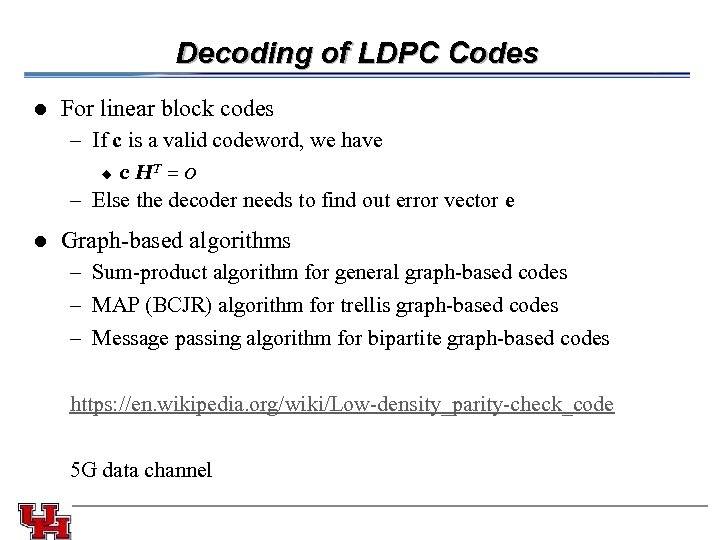Decoding of LDPC Codes l For linear block codes – If c is a valid codeword, we have u c HT = 0 – Else the decoder needs to find out error vector e l Graph-based algorithms – Sum-product algorithm for general graph-based codes – MAP (BCJR) algorithm for trellis graph-based codes – Message passing algorithm for bipartite graph-based codes https: //en. wikipedia. org/wiki/Low-density_parity-check_code 5 G data channel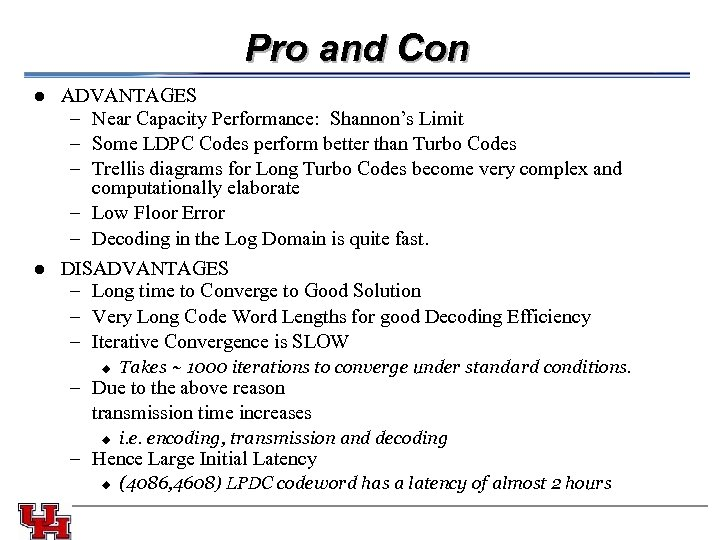Pro and Con l ADVANTAGES – Near Capacity Performance: Shannon’s Limit – Some LDPC Codes perform better than Turbo Codes – Trellis diagrams for Long Turbo Codes become very complex and computationally elaborate – Low Floor Error – Decoding in the Log Domain is quite fast. DISADVANTAGES – Long time to Converge to Good Solution – Very Long Code Word Lengths for good Decoding Efficiency – Iterative Convergence is SLOW l u Takes ~ 1000 iterations to converge under standard conditions. – Due to the above reason transmission time increases u i. e. encoding, transmission and decoding – Hence Large Initial Latency u (4086, 4608) LPDC codeword has a latency of almost 2 hours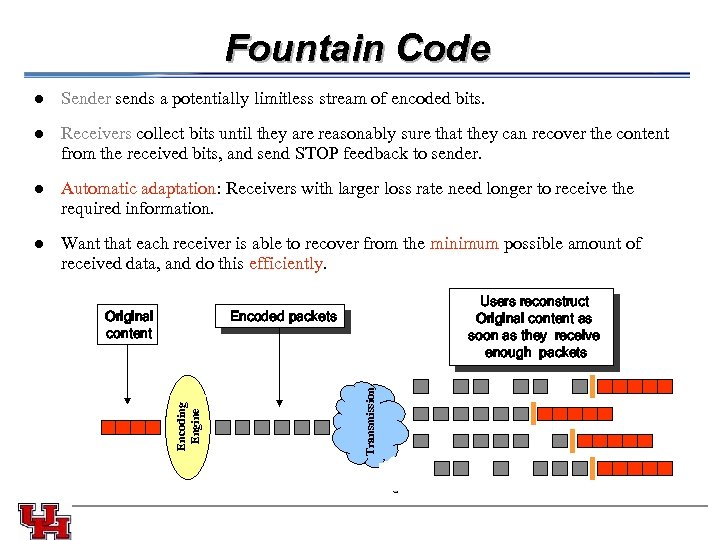Fountain Code l l Automatic adaptation: Receivers with larger loss rate need longer to receive the required information. l Want that each receiver is able to recover from the minimum possible amount of received data, and do this efficiently. Original content Users reconstruct Original content as soon as they receive enough packets Transmission Encoded packets Encoding Engine Receivers collect bits until they are reasonably sure that they can recover the content from the received bits, and send STOP feedback to sender. l Sender sends a potentially limitless stream of encoded bits.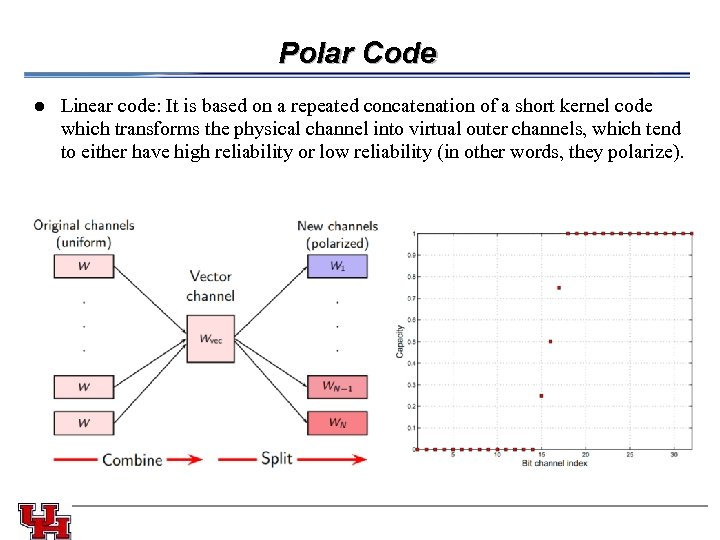Polar Code l Linear code: It is based on a repeated concatenation of a short kernel code which transforms the physical channel into virtual outer channels, which tend to either have high reliability or low reliability (in other words, they polarize).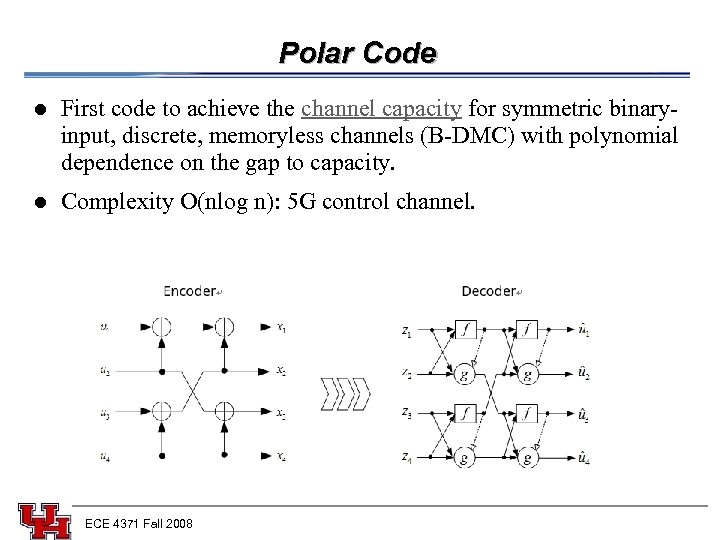Polar Code l l First code to achieve the channel capacity for symmetric binaryinput, discrete, memoryless channels (B-DMC) with polynomial dependence on the gap to capacity. Complexity O(nlog n): 5 G control channel. ECE 4371 Fall 2008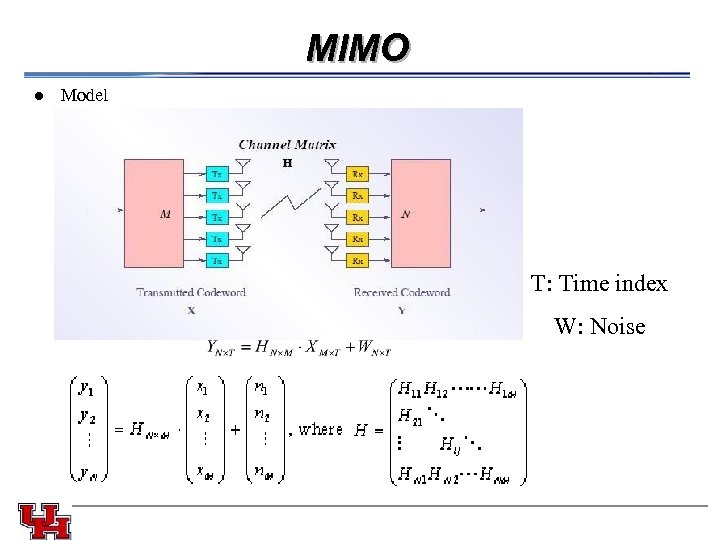MIMO l Model T: Time index W: Noise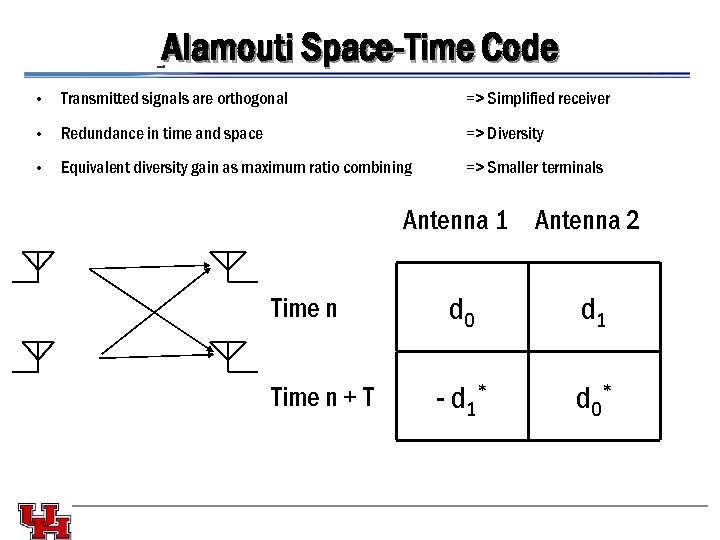Alamouti Space-Time Code • Transmitted signals are orthogonal => Simplified receiver • Redundance in time and space => Diversity • Equivalent diversity gain as maximum ratio combining => Smaller terminals Antenna 1 Antenna 2 Time n + T d 0 d 1 - d 1* d 0 *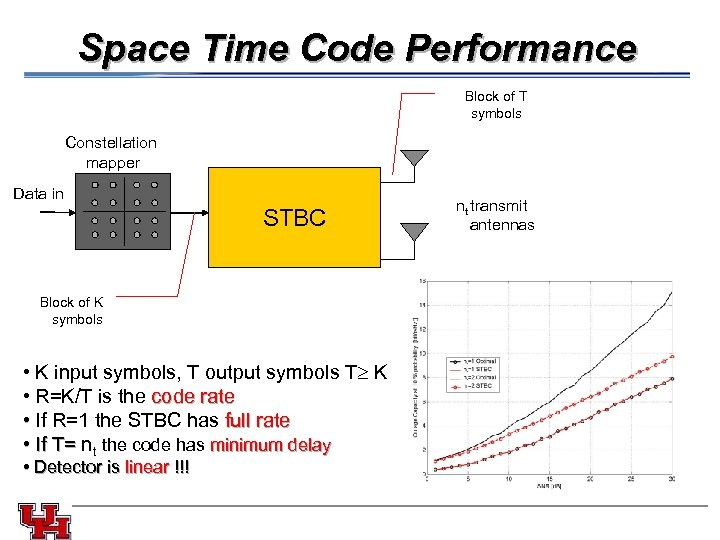Space Time Code Performance Block of T symbols Constellation mapper Data in STBC Block of K symbols • K input symbols, T output symbols T K • R=K/T is the code rate • If R=1 the STBC has full rate • If T= nt the code has minimum delay If T= • Detector is linear !!! nt transmit antennas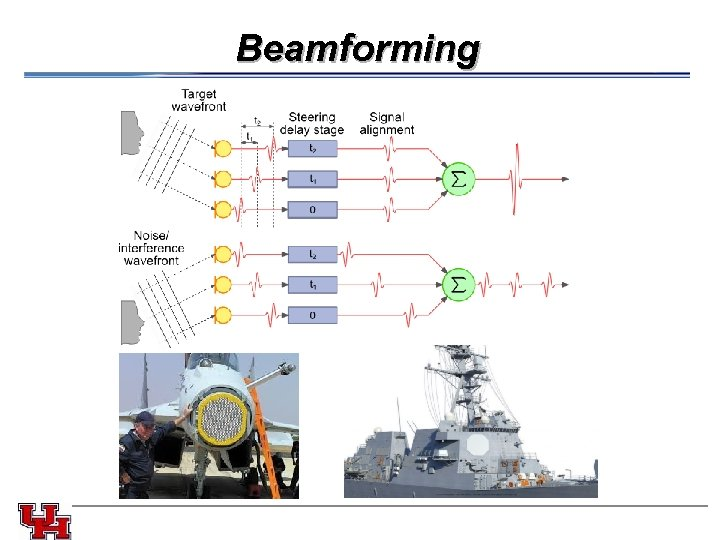Beamforming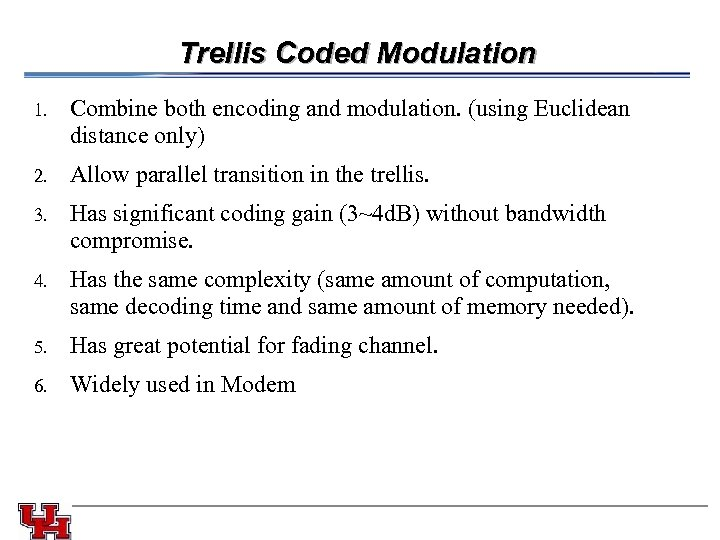Trellis Coded Modulation 1. 2. Allow parallel transition in the trellis. 3. Combine both encoding and modulation. (using Euclidean distance only) Has significant coding gain (3~4 d. B) without bandwidth compromise. 4. Has the same complexity (same amount of computation, same decoding time and same amount of memory needed). 5. Has great potential for fading channel. 6. Widely used in Modem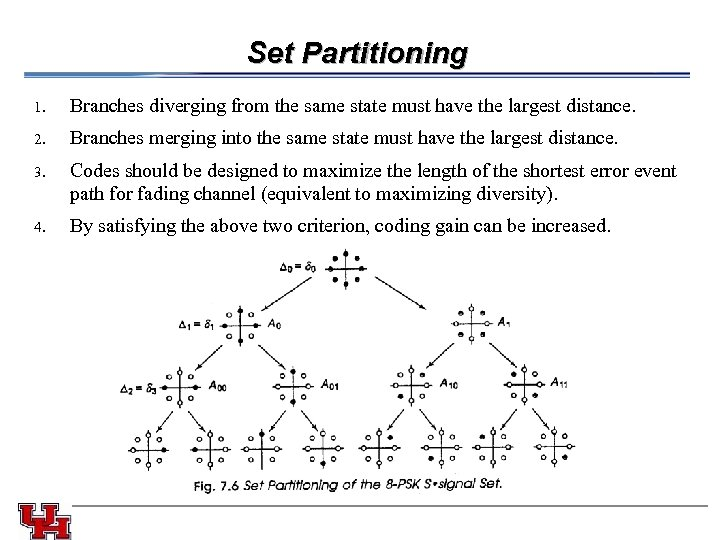Set Partitioning 1. 2. Codes should be designed to maximize the length of the shortest error event path for fading channel (equivalent to maximizing diversity). 4. Branches merging into the same state must have the largest distance. 3. Branches diverging from the same state must have the largest distance. By satisfying the above two criterion, coding gain can be increased.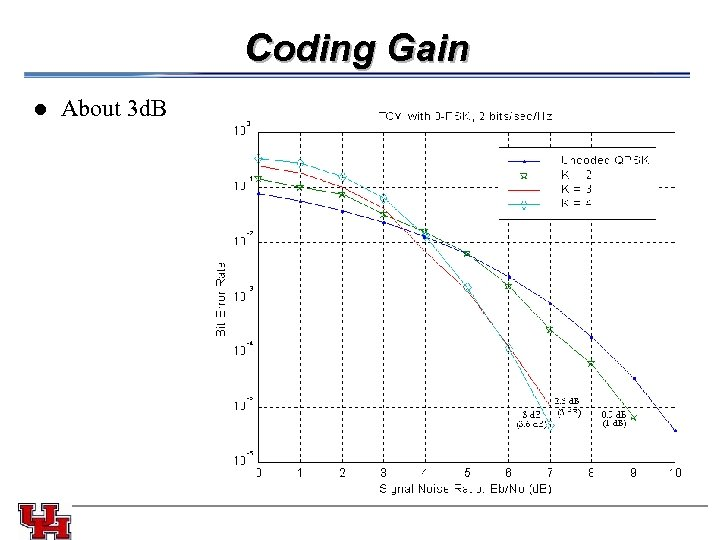Coding Gain l About 3 d. B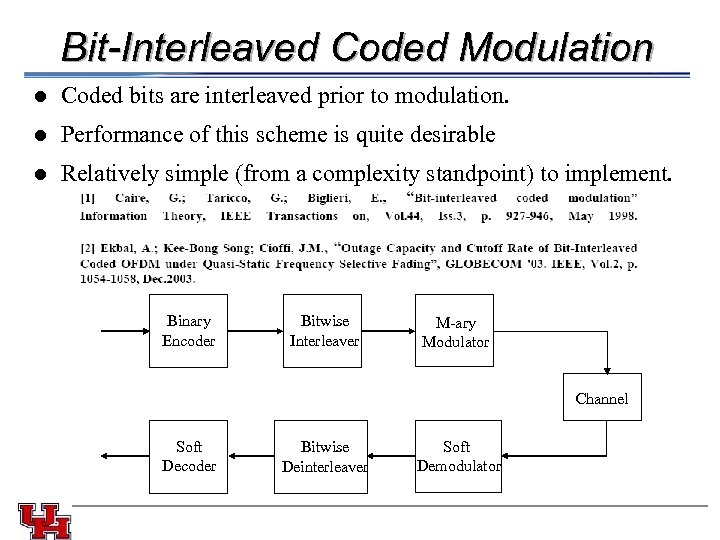Bit-Interleaved Coded Modulation l l Performance of this scheme is quite desirable l Coded bits are interleaved prior to modulation. Relatively simple (from a complexity standpoint) to implement. Binary Encoder Bitwise Interleaver M-ary Modulator Channel Soft Decoder Bitwise Deinterleaver Soft Demodulator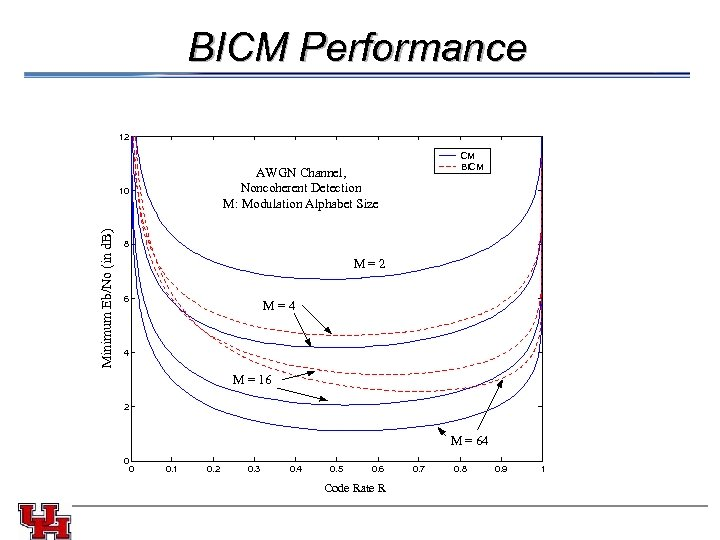BICM Performance 12 AWGN Channel, Noncoherent Detection M: Modulation Alphabet Size Minimum Eb/No (in d. B) 10 CM BICM 8 M = 2 6 M = 4 4 M = 16 2 M = 64 0 0 0. 1 0. 2 0. 3 0. 4 0. 5 0. 6 Code Rate R 0. 7 0. 8 0. 9 1